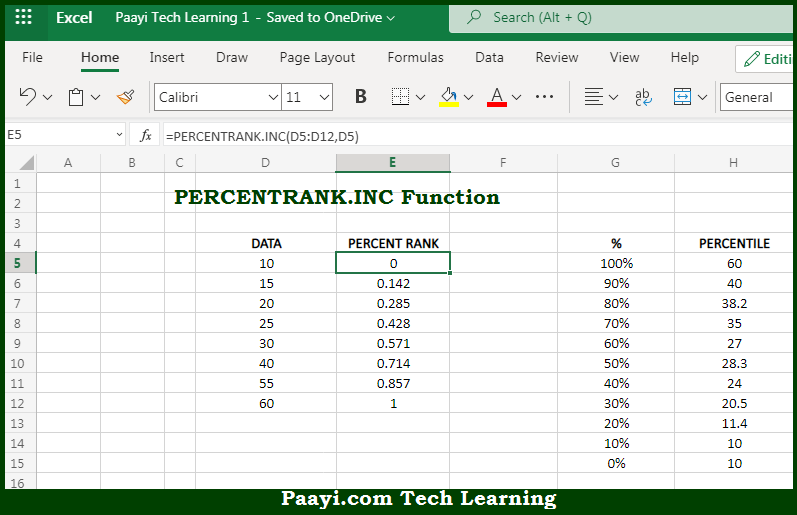# Learn How to Use Microsoft Excel PERCENTRANK.INC Function

Written by | 0 Comments | 443 Views

In this article, you will learn how to use the Microsoft Excel PERCENTRANK.INC function and its prime function in Microsoft Excel. You will also get to know the Microsoft Excel PERCENTRANK.INC function return value and syntax with the help of some example

Microsoft Excel PERCENTRANK.INC Function

The main purpose of the Microsoft Excel PERCENTRANK.INC function is to get the percentile rank, inclusive. That implies, with the help of PERCENTRANK.INC function you can able to return the relative rank of a value in a given data set as a percentage representing the number of values less than or equal to the value. The percentile rank is commonly used as a way to interpret standing in standardized tests. So, with the help of PERCENTRANK.INC function, you can able to get the percentile rank, inclusive.

Return Value of PERCENTRANK.INC Function

The return value will be the calculated rank in the decimal value.

Syntax of PERCENTRANK.INC Function

=PERCENTRANK.INC(array, x, [significance])

Where the arguments:

• array: This is the data value array.
• x: This is the value to rank.
• significance: This is the number of significant digits in the result. Defaults to 3 (optional).

## How to Use Microsoft Excel PERCENTRANK.INC Function?So we know that, Microsoft Excel PERCENTRANK.INC function you can able to get the percentile rank, inclusive. That implies, with the help of PERCENTRANK.INC function you can able to return the relative rank of a value in a given data set as a percentage representing the number of values less than or equal to the value. The percentile rank is commonly used as a way to interpret standing in standardized tests. So, with the help of PERCENTRANK.INC function, you can able to get the percentile rank, inclusive.